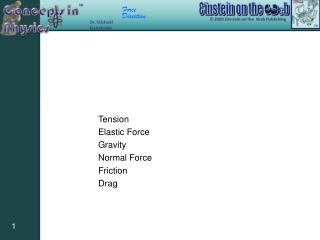Download PresentationTension

# Tension

Télécharger la présentation## Tension

- - - - - - - - - - - - - - - - - - - - - - - - - - - E N D - - - - - - - - - - - - - - - - - - - - - - - - - - -
##### Presentation Transcript

1. Tension Elastic Force Gravity Normal Force Friction Drag

2. Tension The magnitude of the tension is the same everywhere in the rope.

3. Tension If an object that is experiencing the force is pulling but not stretching another object On the object that is experiencing the force at the point where the pulling occurs Determined using Newton’s Second Law Toward the center of the object being pulled (rope, wire, etc.) No associated energy

4. Spring Force

5. Spring Force If an object that is experiencing the force is stretching (or compressing) another object On the object experiencing the force where it connects to the object that stretches/compresses Toward the center of the object that stretches or away from the center of the object that compresses Elastic Potential Energy

6. Gravity

7. Gravity or If there are two massive objects present At the center-of-mass of the object receiving the force or Toward the center of mass of the other massive object Gravitational Potential Energy

8. N m Normal Force N m w

9. Normal Force or If an object touches the surface of another object On the object receiving the force at the point(s) where it touches the surface Determined using Newton’s Second Law Perpendicular to the surface and from the object exerting the force to the object experiencing it No associated energy

10. N f m Direction of motion or possible motion Friction N f m Direction of motion or possible motion

11. Friction or If an object touches the surface of another object On the object receiving the force at the point(s) where it touches the surface or In a direction that opposes the motion or the possibility of motion Thermal Energy

12. Drag D

13. Drag If an object is moving through a liquid or gas. On the object receiving the force at the point(s) where it touches the liquid or gas In a direction that opposes the motion Thermal Energy